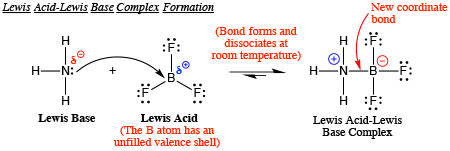# Question #1628a

Jul 30, 2017

The B-I bond is weaker than the B-Br bond.

#### Explanation:

Because the bond between B and I is weaker, that means $B {I}_{3}$ is easier to dissociate than $B B {r}_{3}$. Because it is easier to dissociate, $B {I}_{3}$ is a stronger acid.

Oct 7, 2017

${\text{BI}}_{3}$ has a longer $\text{B—X}$ σ bond.

#### Explanation:

The boron halides are Lewis acids. Their acid strength depends on their ability to accept a pair of electrons from a Lewis base.Thus, we might expect ${\text{BF}}_{3}$, with its highly electronegative $\text{F}$ atoms, to be the strongest acid of the boron halides.

However, it is the weakest Lewis acid of the group.

It used to be thought that the reason involved overlap between the vacant $\text{2p}$ orbital of $\text{B}$ and the filled $\text{p}$ orbital of the halogen (π bonding).

Recent research suggests that σ bonding, not π bonding, is the important factor.

In ${\text{BF}}_{3}$, the $\text{B—F}$ bond distance is short.

The ${\text{sp}}^{2}$ orbitals of $\text{B}$ and the $\text{2p}$ orbitals of $\text{F}$ are about the same size.

Thus, there is good $\text{sp"^2"-2p}$ overlap (σ bonding) between the $\text{B}$ and $\text{F}$ atoms.

This increases the electron density on the $\text{B}$ atom and decreases its Lewis acidity.

In ${\text{BI}}_{3}$, the $\text{B—I}$ bond length is longer and the $\text{I}$ atom is using a much larger $\text{5p}$ orbital to form the σ bond.

Thus, the $\text{sp"^2"-5p}$ orbital overlap is quite small.

Since the σ electrons around $\text{B}$ are much further away, the electron density is greatly reduced.

${\text{BI}}_{3}$ is therefore the strongest Lewis acid.

The order of acidity is

${\text{BI"_3 >"BBr"_3 > "BCl"_3 > "BF}}_{3}$